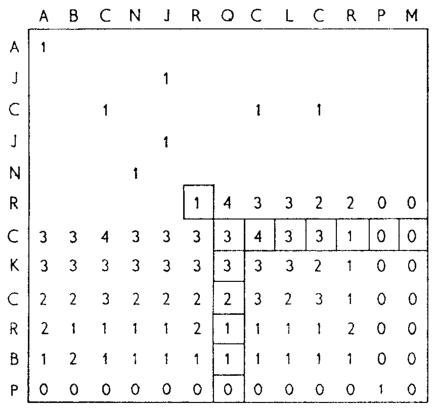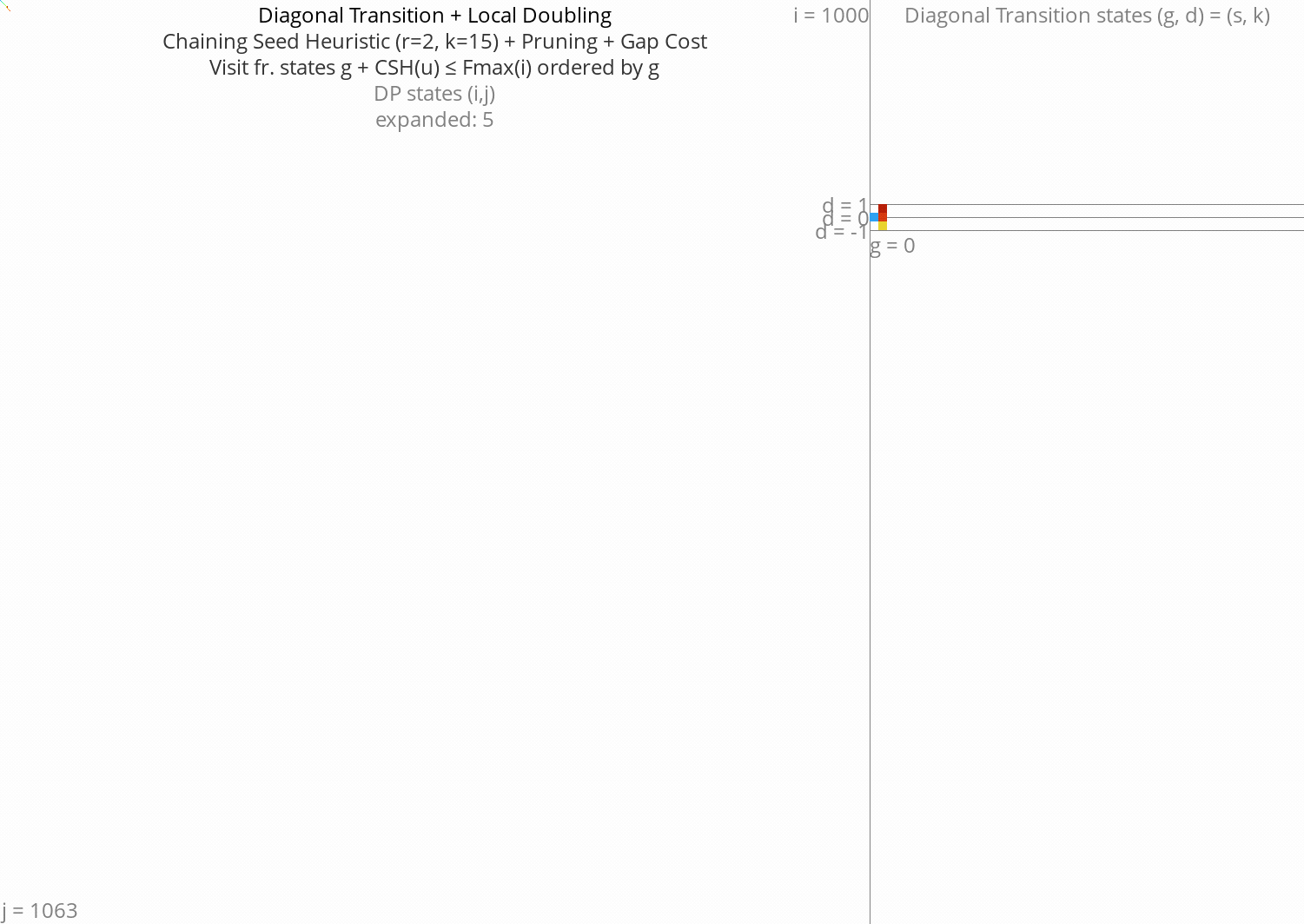# Local Doubling

\begin{equation*} \newcommand{\st}{\langle #1,#2\rangle} \newcommand{\g}{g^*} \newcommand{\fm}{f_{max}} \newcommand{\gap}{\operatorname{Gap}} \end{equation*}

This post is an overview/review of existing alignment algorithms, explained using visualizations. This builds up to the introduction of local doubling, an idea I had while writing a previous post on pre-pruning. My hope is that this can combine the efficiency of Needleman-Wunsch or Diagonal Transition implementations (e.g. Edlib and WFA using bitpacking and SIMD), while having the better complexity of A*-based alignment.

See also my review post on pairwise alignment, comparing algorithms and their runtime/memory trade-offs. This post aims to be visual and intuitive, while that one (as for now) is more formal/technical.

## Notation

• Input: sequences of length $$n$$ and $$m$$. For complexity analysis, we assume that $$m=n$$.
• States of the alignment graph:
• states $$u = \st ij$$,
• start $$s = \st 00$$,
• end $$t = \st nm$$.
• Distances:
• distance between vertices $$d(u, v)$$,
• distance from start $$g = g(u) := d(s, u)$$,
• gapcost: $$\gap(u, v) = \gap(\st ij, \st {i’}{j’}) = |(i’-i) - (j’-j)|$$.

## Needleman-Wunsch: where it all begins

• Invented by (Needleman and Wunsch 1970) and others. See the review post.
• Visits all states $$\st ij$$ of the DP table ordered by column $$i$$.
• In fact, any topological sort (total order) of the states of the underlying dependency graph (partially ordered set) is allowed. One particular optimization is process anti-diagonals in order. Those correspond to anti-chains in the poset, and hence can be computed independently of each other.
• Complexity: $$O(n^2)$$.Figure 1: NW expands all 1000x1000 states. Ignore the right half for now.

## Dijkstra/BFS: visiting fewer states

• Mentioned in Ukkonen (1985)

• Visit states ordered by the distance from start $$s$$: $$g(u) := d(s, u)$$.

• Visits exactly those states with

\begin{equation} g(u) \leq \g,\label{dijsktra} \end{equation}

where $$\g := d(s, t)$$ is the total alignment distance.

• Drawback: much slower:

• bithacks/SIMD don’t work,
• queues are slow compared to iteration.
• Complexity: $$O(n d)$$, where $$d=\g$$ is the edit distance.

• Typical notation is $$O(ns)$$ for arbitrary scores and $$O(nd)$$ for edit distance. I’d use $$O(n\g)$$ for consistency but it’s ugly and non-standard.

## Band doubling: Dijkstra, but more efficient

• Introduced in Ukkonen (1985)
• Idea: use NW’s efficient column-order to compute only the states with $$g(u) \leq \g$$.
• We don’t know $$\g$$, but we can find it using exponential search or band doubling.
• For a guess $$\fm$$ of $$\g$$:
• Compute all states with

\begin{equation} \gap(s, u) \leq \fm,\label{doubling} \end{equation}

where $$\gap(s, \st ij)$$ is the cost of indels (gapcost) needed to go from $$s$$ to $$\st ij$$. For edit distance this is simply $$|i-j|$$.

• When $$g(t) \leq \fm$$, all states with $$g\leq \g =g(t) \leq \fm$$ have been computed, and we found an optimal path.

• Otherwise, double the value of $$\fm$$ and try again.

• Starting with $$\fm = 1$$, this takes $$T:=1n + 2n + \dots + f_{last}\cdot n$$ time. Since $$f_{last}/2 < \g$$ we have $$T= (2f_{last}-1)n \leq 4\g n$$.
• Complexity: $$O(nd)$$

## GapCost: A first heuristic

• We can sharpen \eqref{doubling} by also bounding the indel cost (gapcost) from $$u$$ to the end:

\begin{equation} \gap(s, u)+\gap(u, t) \leq \fm,\label{doubling-gap} \end{equation}

• Assuming both input sequences are the same length ($$m=n$$), this halves the runtime.

• This can also be used on top of Dijkstra to give a first A* variant where states are ordered by $$f(u) := g(u) + \gap(u, t)$$.

• It is possible to transform the insertion and deletion costs in a way that already accounts for the gapcost, see this post.

• Introduced in Spouge (1989)

• Equations \eqref{doubling} and \eqref{doubling-gap} determine the area to be computed up-front. But we can make a simple improvement and take into account the current distance $$g(u) \geq \gap(s, u)$$:

\begin{equation} g(u)+\gap(u, t) \leq \fm.\label{volume-gap} \end{equation}

An even simpler option is $$g(u) \leq \fm$$, which corresponds directly to computing increasing portions of Dijkstra.

• This still relies on repeated doubling of $$\fm$$.

## Cheating: an oracle gave us $$g^*$$

• If we already know the target distance $$\g$$, we can skip the exponential search over $$\fm$$ and directly use $$\fm = \g$$. This will speed up all of the band doubling algorithms above up to $$4$$ times:
• no need to try smaller $$\fm<\g$$ => $$2x$$ faster,
• no more unlucky cases where $$\fm=2\g-\epsilon$$.
• More generally, we can make an initial guess for $$\fm$$ if we roughly know the distance distribution of the input.

## A*: Better heuristics

• Instead of visiting states by column $$i$$ or distance $$g$$, we can order by

\begin{equation} f(u) := g(u)+h(u) \leq \g,\label{astar} \end{equation}

where $$h$$ is any heuristic function satisfying $$h(u) \leq d(u, t)$$.

• Drawback: Again, A* is slow because of the priority queue and many computations of $$h$$.

## Broken idea: A* and computational volumes

• Just like band doubling speeds up Dijkstra, can we use it to speed up A*?
• Start with $$\fm = h(s)$$.
• Compute all states with $$f(u) \leq \fm$$ in column-order.
• Double $$\fm$$ after each try.
• BROKEN: If we start with $$\fm = h(s) = \g-1$$ and we double to $$\fm = 2\g-2$$ the number of expanded states goes from $$O(n)$$ to $$O(n^2)$$.

## Goal: Diagonal Transition + pruning + local doubling

Here’s a gif with pruning as well:Figure 13: DT + CSH + gapcost + pruning + Local-Doubling

## Cheating more: an oracle gave us the optimal path

• Pruning brings a challenge to the local

## TODO: aspriation windows

In chess engines (ie alpha beta search/pruning) there is the concept of aspiration window which is similar to exponential search. Maybe we can reuse some concepts.

Needleman, Saul B., and Christian D. Wunsch. 1970. “A General Method Applicable to the Search for Similarities in the Amino Acid Sequence of Two Proteins.” Journal of Molecular Biology 48 (3): 443–53. https://doi.org/10.1016/0022-2836(70)90057-4.
Spouge, John L. 1989. “Speeding up Dynamic Programming Algorithms for Finding Optimal Lattice Paths.” Siam Journal on Applied Mathematics 49 (5): 1552–66. https://doi.org/10.1137/0149094.
Ukkonen, Esko. 1985. “Algorithms for Approximate String Matching.” Information and Control 64 (1–3): 100–118. https://doi.org/10.1016/s0019-9958(85)80046-2.